# 【深度学习基础】第十六课：指数加权平均

## 指数加权平均，偏差修正

Posted by x-jeff on February 25, 2020

【深度学习基础】系列博客为学习Coursera上吴恩达深度学习课程所做的课程笔记。

# 1.指数加权平均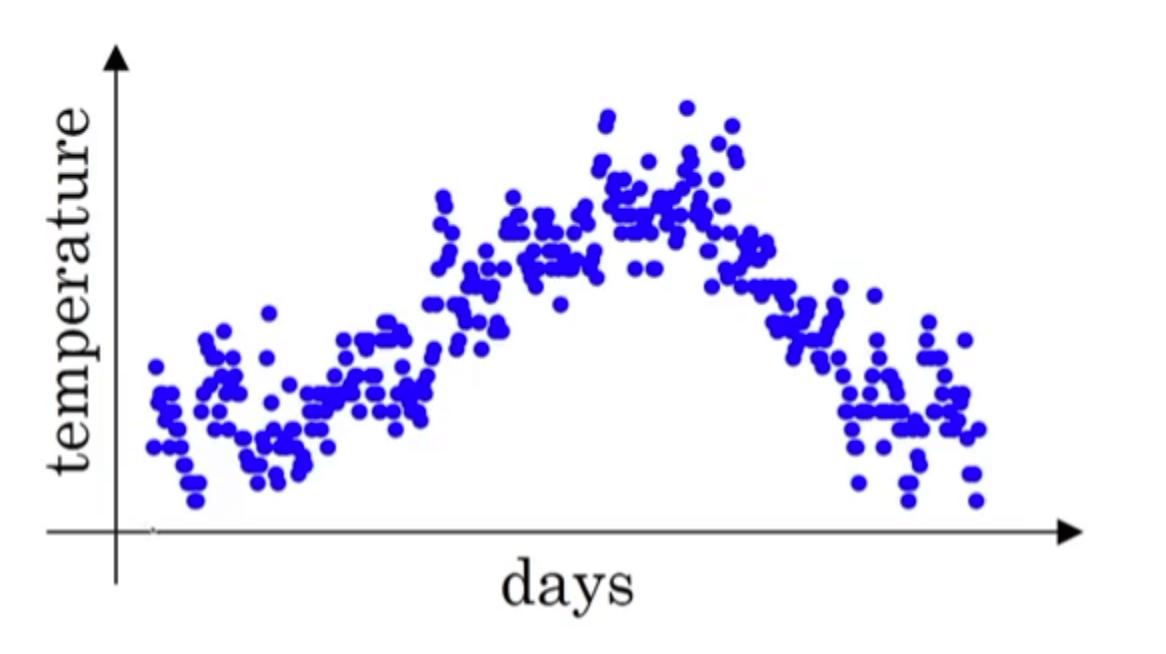• $V_0=0$
• $V_1=0.9V_0+0.1\theta_1$
• $V_2=0.9V_1+0.1\theta_2$
• $V_3=0.9V_2+0.1\theta_3$
• ……
• $V_t=0.9V_{t-1}+0.1\theta_t$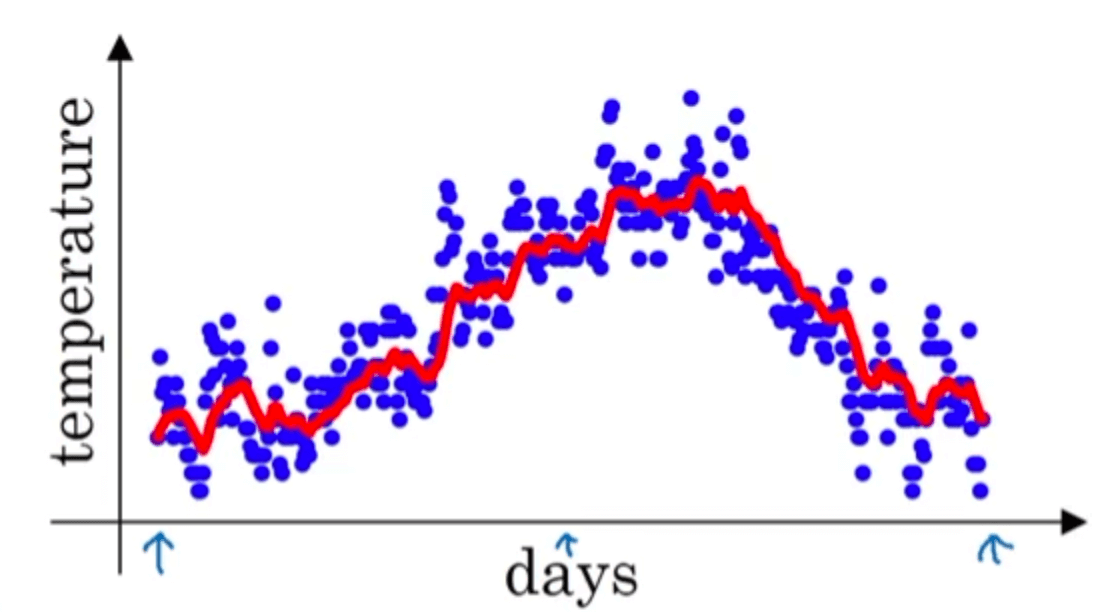$V_t=\beta V_{t-1}+(1-\beta) \theta_t$

👉如果有$\beta=0.98$，会得到下图的绿线：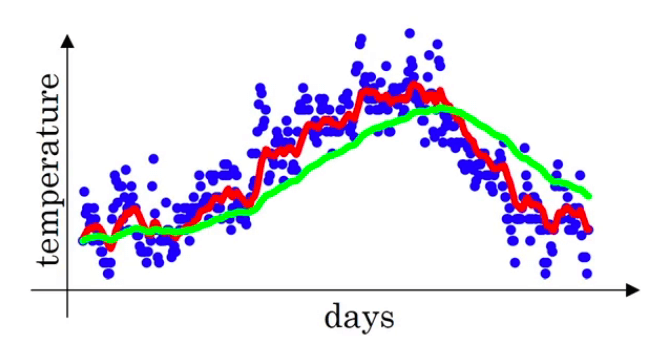👉如果有$\beta=0.5$，会得到下图的黄线：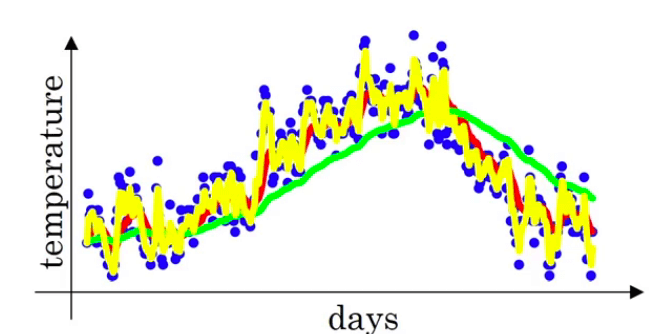# 2.指数加权平均的作用

• $V_{100}=0.9V_{99}+0.1\theta_{100}$
• $V_{99}=0.9V_{98}+0.1\theta_{99}$
• $V_{98}=0.9V_{97}+0.1\theta_{98}$
• $V_{97}=0.9V_{96}+0.1\theta_{97}$
• ……

\begin{align} V_{100} & = 0.1\theta_{100} + 0.9V_{99} \\ & = 0.1\theta_{100} + 0.1*0.9\theta_{99} + 0.9^2V_{98} \\ & = 0.1\theta_{100}+0.1*0.9\theta_{99}+0.1*0.9^2\theta_{98}+0.9^3V_{97} \\ & = 0.1\theta_{100}+0.1*0.9\theta_{99}+0.1*0.9^2\theta_{98}+......+0.1*0.9^{99}\theta_1 \end{align}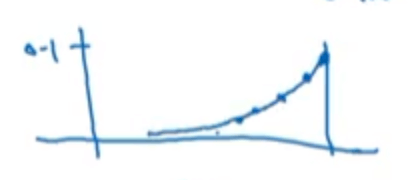$0.1*\frac{1}{e}\approx 0.1* 0.9^{10}$

# 3.偏差修正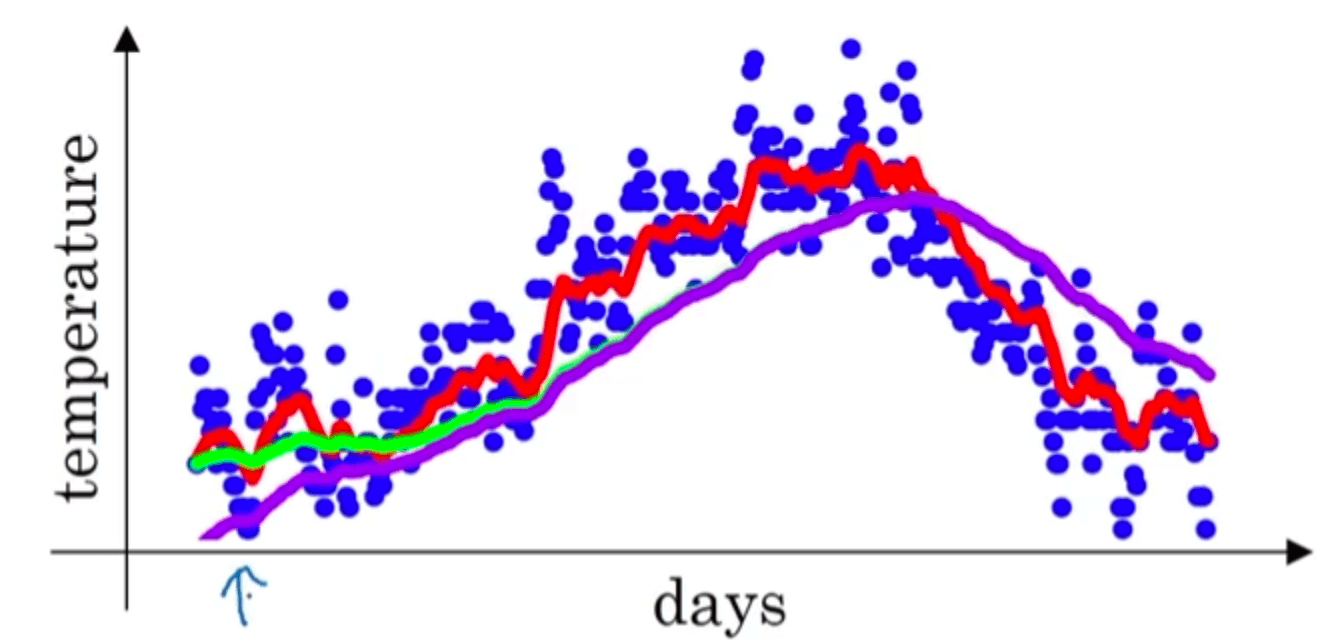• $V_0=0$
• $V_1=0.98V_0+0.02\theta_1=0.02\times 40=0.8$
• $V_2=0.98V_1+0.02\theta_2=0.98\times 0.8+0.02\times 55=1.884$
• ……

$\frac{V_t}{1-\beta^t}$

$V_1$修正之后的结果为($\frac{0.8}{1-0.98^1}=$)40；$V_2$修正之后的结果为($\frac{1.884}{1-0.98^2} \approx$)47.58。很明显，修正之后的结果更为准确。